• Shark源码分析（三）：数据预处理正则化在机器学习算法中，获取训练数据后首先要做的不是将输入投入训练方法中进行学习，而是应该对数据进行预处理。预处理过程输出数据的质量能够对之后算法的结果起着至关重要的...
Shark源码分析（三）：数据预处理之正则化
在机器学习算法中，获取训练数据后首先要做的不是将输入投入训练方法中进行学习，而是应该对数据进行预处理。预处理过程输出数据的质量能够对之后算法的结果起着至关重要的作用。预处理过程含有非常多的操作，在我目前阅读代码的过程中只碰到了正则化这一过程。那我们就先来讨论正则化，如果之后再碰到了其他的方法再补充。
Shark将对输入数据进行正则化的模型也看作是一个线性模型。Shark给出了两种正则化的方法，分别是NormalizeComponentsUnitInterval, NormalizeComponentsUnitVariance。对于这两种不同的正则化方法有两个不同的trainer（联系上一篇博客的内容）进行训练。
第一种方法是将每一维度的特征都缩小到

[0,1]
$[0, 1]$范围内，这对于特征值是有界的情况来说是有效的。而第二种方法是将每一维度的方差都调整到1。对于『将均值变为0』这一操作来说是可选的。如果不包含这一操作，那么这一方法对于高维度的稀疏特征向量来说是有效的。
Normalizer类
该类定义在<include/shark/Models/Normalizer.h>文件中。
其与普通线性模型的不同之处在于：
输入与输出的维度必须是相同的对于每一维度需要单独进行计算
template <class DataType = RealVector>
class Normalizer : public AbstractModel<DataType, DataType>
{
protected:
RealVector m_A; //权值向量
RealVector m_b; //偏置向量                          bool m_hasOffset; //表示是否需要偏置向量
public:
typedef AbstractModel<DataType, DataType> base_type;
typedef Normalizer<DataType> self_type;

typedef typename base_type::BatchInputType BatchInputType;
typedef typename base_type::BatchOutputType BatchOutputType;

Normalizer()
{ }

Normalizer(const self_type& model)
: m_A(model.m_A)
, m_b(model.m_b)
, m_hasOffset(model.m_hasOffset)
{ }

Normalizer(std::size_t dimension, bool hasOffset = false)
: m_A(dimension, dimension)
, m_b(dimension)
, m_hasOffset(hasOffset)
{ }

Normalizer(RealVector diagonal)
: m_A(diagonal)
, m_hasOffset(false)
{ }

Normalizer(RealVector diagonal, RealVector vector)
: m_A(diagonal)
, m_b(vector)
, m_hasOffset(true)
{ }

std::string name() const
{ return "Normalizer"; }

friend void swap(const Normalizer& model1, const Normalizer& model2)
{
std::swap(model1.m_A, model2.m_A);
std::swap(model1.m_b, model2.m_b);
std::swap(model1.m_hasOffset, model2.m_hasOffset);
}

const self_type operator = (const self_type& model)
{
m_A = model.m_A;
m_b = model.m_b;
m_hasOffset = model.m_hasOffset;
}

boost::shared_ptr<State> createState() const
{
return boost::shared_ptr<State>(new EmptyState());
}

//判断模型是否有被正确地初始化
bool isValid() const
{
return (m_A.size() != 0);
}

bool hasOffset() const
{
return m_hasOffset;
}

//返回权值向量
RealVector const& diagonal() const
{
SHARK_CHECK(isValid(), "[Normalizer::matrix] model is not initialized");
return m_A;
}

//返回偏置向量
RealVector const& offset() const
{
SHARK_CHECK(isValid(), "[Normalizer::vector] model is not initialized");
return m_b;
}

std::size_t inputSize() const
{
SHARK_CHECK(isValid(), "[Normalizer::inputSize] model is not initialized");
return m_A.size();
}

std::size_t outputSize() const
{
SHARK_CHECK(isValid(), "[Normalizer::outputSize] model is not initialized");
return m_A.size();
}

//将权值向量与偏置向量合在一起进行输出
RealVector parameterVector() const
{
SHARK_CHECK(isValid(), "[Normalizer::parameterVector] model is not initialized");
std::size_t dim = m_A.size();
if (hasOffset())
{
RealVector param(2 * dim);
init(param)<<m_A,m_b;
return param;
}
else
{
RealVector param(dim);
init(param)<<m_A;
return param;
}
}

//可以更改权值向量与偏置向量的值
void setParameterVector(RealVector const& newParameters)
{
SHARK_CHECK(isValid(), "[Normalizer::setParameterVector] model is not initialized");
std::size_t dim = m_A.size();
if (hasOffset())
{
SIZE_CHECK(newParameters.size() == 2 * dim);
init(newParameters)>>m_A,m_b;
}
else
{
SIZE_CHECK(newParameters.size() == dim);
init(newParameters)>>m_A;
}
}

std::size_t numberOfParameters() const
{
SHARK_CHECK(isValid(), "[Normalizer::numberOfParameters] model is not initialized");
return (m_hasOffset) ? m_A.size() + m_b.size() : m_A.size();
}

//在训练完成之后，将权值向量与偏置向量保存到模型中
void setStructure(RealVector const& diagonal)
{
m_A = diagonal;
m_hasOffset = false;
}

void setStructure(std::size_t dimension, bool hasOffset = false)
{
m_A.resize(dimension);
m_hasOffset = hasOffset;
if (hasOffset) m_b.resize(dimension);
}

void setStructure(RealVector const& diagonal, RealVector const& offset)
{
SHARK_CHECK(diagonal.size() == offset.size(), "[Normalizer::setStructure] dimension conflict");
m_A = diagonal;
m_b = offset;
m_hasOffset = true;
}

using base_type::eval;

//对输入利用权值向量以及偏置向量进行正则化
void eval(BatchInputType const& input, BatchOutputType& output) const
{
SHARK_CHECK(isValid(), "[Normalizer::eval] model is not initialized");
output.resize(input.size1(), input.size2());
noalias(output) = input * repeat(m_A,input.size1());
if (hasOffset())
{
noalias(output) += repeat(m_b,input.size1());
}
}

void eval(BatchInputType const& input, BatchOutputType& output, State& state) const
{
eval(input, output);
}

{
archive & m_A;
archive & m_b;
archive & m_hasOffset;
}

void write(OutArchive& archive) const
{
archive & m_A;
archive & m_b;
archive & m_hasOffset;
}
};
注意到这个类也是继承自AbstractModel。
正则化模型的使用方式是，利用输入的训练数据训练正则化模型的参数。如果之后有测试数据输入进来，可以使用同样的模型对测试数据进行正则化，而不需要再重新训练模型。
NormalizeComponentsUnitVariance类
该类定义在<include/shark/Algorithms/Trainers/NormalizeComponentsUnitVariance.h>文件中。
在这个方法中，零均值化默认是被关闭的。因为对输入是一个稀疏矩阵的情况来说，零均值话是会破坏它的稀疏性。如果当输入数据不是稀疏的话，可以开启这一项。
其正则化方法是

x′=x−μσ=1σ⋅x−μσ
$x' = \frac{x - \mu}{\sigma} = \frac{1}{\sigma} \cdot x - \frac{\mu}{\sigma}$
template <class DataType = RealVector>
class NormalizeComponentsUnitVariance : public AbstractUnsupervisedTrainer< Normalizer<DataType> >
{
public:
typedef AbstractUnsupervisedTrainer< Normalizer<DataType> > base_type;

NormalizeComponentsUnitVariance(bool zeroMean)
: m_zeroMean(zeroMean){ }

std::string name() const
{ return "NormalizeComponentsUnitVariance"; }

void train(Normalizer<DataType>& model, UnlabeledData<DataType> const& input)
{
SHARK_CHECK(input.numberOfElements() >= 2, "[NormalizeComponentsUnitVariance::train] input needs to consist of at least two points");

RealVector mean;
RealVector variance;
meanvar(input, mean, variance); //计算数据的均值，方差

RealVector diagonal(dc);
RealVector vector(dc);

for (std::size_t d=0; d != dc; d++){
double stddev = std::sqrt(variance(d));
if (stddev == 0.0)
{
diagonal(d) = 0.0;
vector(d) = 0.0;
}
else
{
diagonal(d) = 1.0 / stddev;
vector(d) = -mean(d) / stddev;
}
}

if (m_zeroMean)
model.setStructure(diagonal, vector);
else
model.setStructure(diagonal);
}

protected:
bool m_zeroMean;
};
注意到该方法是继承自AbstractUnsupervisedTrainer，也把它作为无监督学习方法。
NormalizeComponentsUnitInterval类
该类定义在<include/shark/Algorithms/Trainers/NormalizeComponentsUnitInterval.h>文件中。
该方法是将数据的范围都变换到

[0,1]
$[0,1]$区间内，这样做的话势必会破坏数据的稀疏性，所以可能会偏向于选择NormalizeComponentsUnitVariance这一方法。
该方法的公式是

x′=x−minmax−min
$x' = \frac{x - min}{max - min}$
template <class DataType = RealVector>
class NormalizeComponentsUnitInterval : public AbstractUnsupervisedTrainer< Normalizer<DataType> >
{
public:
typedef AbstractUnsupervisedTrainer< Normalizer<DataType> > base_type;

NormalizeComponentsUnitInterval()
{ }

std::string name() const
{ return "NormalizeComponentsUnitInterval"; }

void train(Normalizer<DataType>& model, UnlabeledData<DataType> const& input)
{
std:: size_t ic = input.numberOfElements();
SHARK_CHECK(ic >= 2, "[NormalizeComponentsUnitInterval::train] input needs to consist of at least two points");

//取出每一维度的第一个数据
//求出每一维度的最大值和最小值
RealVector min = input.element(0);
RealVector max = input.element(0);
for(std::size_t i=1; i != ic; i++){
for(std::size_t d = 0; d != dc; d++){
double x = input.element(i)(d);
min(d) = std::min(min(d), x);
max(d) = std::max(max(d), x);
}
}

RealVector diagonal(dc);
RealVector offset(dc);

for (std::size_t d=0; d != dc; d++)
{
if (min(d) == max(d)) //这一维数据的值相同
{
diagonal(d) = 0.0;
offset(d) = -min(d) + 0.5;//偏移设置成0.5就好
}
else
{
double n = 1.0 / (max(d) - min(d));
diagonal(d) = n;
offset(d) = -min(d) * n;
}
}

model.setStructure(diagonal, offset);
}
};
展开全文shark
• 机器学习中几乎都可以看到损失函数后面会添加一个额外项，常用的额外项一般有两种，一般英文称作L1-norm 和 L2 -norm，中文称作 L1正则化 和 L2正则化，或者 L1范数 和 L2范数 L1正则化和L2正则化可以看做是损失函数...
正则化的目的 平衡训练误差与模型复杂度的一种方式，通过加入正则项来避免过拟合（over-fitting）
结构风险最小化（SRM）理论　 经验风险最小化 + 正则化项 = 结构风险最小化
经验风险最小化（ERM），是为了让拟合的误差足够小，即：对训练数据的预测误差很小。但是，我们学习得到的模型，当然是希望对未知数据有很好的预测能力（泛化能力），这样才更有意义。当拟合的误差足够小的时候，可能是模型参数较多，模型比较复杂，此时模型的泛化能力一般。于是，我们增加一个正则化项，它是一个正的常数乘以模型复杂度的函数，aJ(f)，a>=0 用于调整ERM与模型复杂度的关系。结构风险最小化（SRM），相当于是要求拟合的误差足够小，同时模型不要太复杂（正则化项的极小化），这样得到的模型具有较强的泛化能力。
机器学习中几乎都可以看到损失函数后面会添加一个额外项，常用的额外项一般有两种，一般英文称作L1-norm 和 L2 -norm，中文称作 L1正则化 和 L2正则化，或者 L1范数 和 L2范数 L1正则化和L2正则化可以看做是损失函数的惩罚项。所谓『惩罚』是指对损失函数中的某些参数做一些限制。对于线性回归模型，使用L1正则化的模型建叫做Lasso回归，使用L2正则化的模型叫做Ridge回归（岭回归）。下图是Python中Lasso回归的损失函数，式中加号后面一项α∣∣w∣∣1， 即为L1正则化项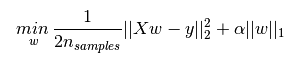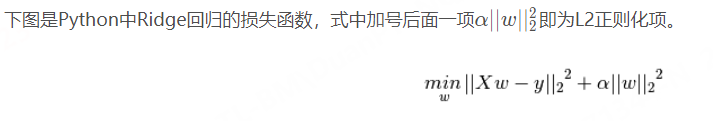一般回归分析中w 表示特征的系数，从上式可以看到正则化项是对系数做了处理（限制）。L1正则化和L2正则化的说明如下：
L1正则化是指权值向量w中各个元素的绝对值之和
L2正则化是指权值向量w 中各个元素的平方和然后再求平方根（可以看到Ridge回归的L2正则化项有平方符号） ​  一般都会在正则化项之前添加一个系数，Python的机器学习包sklearn中用α 表示，一些文章也用λ 表示。这个系数，其中λ越大表明惩罚粒度越大，等于0表示不做惩罚，需要用户指定。
那添加L1和L2正则化有什么用？下面是L1正则化和L2正则化的比较：
L1正则化可以产生稀疏权值矩阵，即产生一个稀疏模型，可以用于特征选择L2正则化可以防止模型过拟合（overfitting）；一定程度上，L1也可以防止过拟合L1会趋向于产生少量的特征，而其他的特征都是0，而L2会选择更多的特征，这些特征都会接近于0。Lasso在特征选择时候非常有用，而Ridge就只是一种规则化而已。ElasticNet 吸收了两者的优点，当有多个相关的特征时，Lasso 会随机挑选他们其中的一个，而ElasticNet则会选择两个；并且ElasticNet 继承 Ridge 的稳定性.
总结： 结构风险最小化是一种模型选择的策略，通过加入正则项以平衡模型复杂度和经验误差；更直观的解释——正则项就是模型参数向量（w）的范数，一般有L1，L2两种常用的范数
参考文章： 7. https://blog.csdn.net/jinping_shi/article/details/52433975
展开全文机器学习
• 这一节我们讲一讲输入数据预处理正则化以及损失函数设定的一些事情。 2. 数据与网络的设定 前一节提到前向计算涉及到的组件(主要是神经元)设定。神经网络结构和参数设定完毕之后，我们就得到得分函数/score
作者：寒小阳  时间：2016年1月。  出处：http://blog.csdn.net/han_xiaoyang/article/details/50451460  声明：版权所有，转载请联系作者并注明出处
1. 引言
上一节我们讲完了各种激励函数的优缺点和选择，以及网络的大小以及正则化对神经网络的影响。这一节我们讲一讲输入数据预处理、正则化以及损失函数设定的一些事情。
2. 数据与网络的设定
前一节提到前向计算涉及到的组件(主要是神经元)设定。神经网络结构和参数设定完毕之后，我们就得到得分函数/score function(忘记的同学们可以翻看一下之前的博文)，总体说来，一个完整的神经网络就是在不断地进行线性映射(权重和input的内积)和非线性映射(部分激励函数作用)的过程。这一节我们会展开来讲讲数据预处理，权重初始化和损失函数的事情。
2.1 数据预处理
在卷积神经网处理图像问题的时候，图像数据有3种常见的预处理可能会用到，如下。我们假定数据表示成矩阵为X，其中我们假定X是[N*D]维矩阵(N是样本数据量，D为单张图片的数据向量长度)。
去均值，这是最常见的图片数据预处理，简单说来，它做的事情就是，对待训练的每一张图片的特征，都减去全部训练集图片的特征均值，这么做的直观意义就是，我们把输入数据各个维度的数据都中心化到0了。使用python的numpy工具包，这一步可以用X -= np.mean(X, axis = 0)轻松实现。当然，其实这里也有不同的做法：简单一点，我们可以直接求出所有像素的均值，然后每个像素点都减掉这个相同的值；稍微优化一下，我们可以在RGB三个颜色通道分别做这件事。归一化，归一化的直观理解含义是，我们做一些工作去保证所有的维度上数据都在一个变化幅度上。通常我们有两种方法来实现归一化。一个是在数据都去均值之后，每个维度上的数据都除以这个维度上数据的标准差(X /= np.std(X, axis = 0))。另外一种方式是我们除以数据绝对值最大值，以保证所有的数据归一化后都在-1到1之间。多说一句，其实在任何你觉得各维度幅度变化非常大的数据集上，你都可以考虑归一化处理。不过对于图像而言，其实这一步反倒可做可不做，因为大家都知道，像素的值变化区间都在[0,255]之间，所以其实图像输入数据天生幅度就是一致的。
上述两个操作对于数据的作用，画成示意图，如下：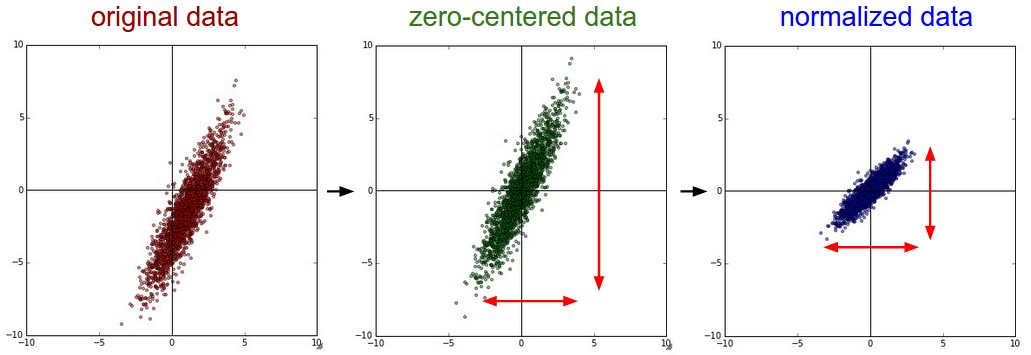PCA和白化/whitening，这是另外一种形式的数据预处理。在经过去均值操作之后，我们可以计算数据的协方差矩阵，从而可以知道数据各个维度之间的相关性，简单示例代码如下：
# 假定输入数据矩阵X是[N*D]维的
X -= np.mean(X, axis = 0) # 去均值
cov = np.dot(X.T, X) / X.shape # 计算协方差
得到的结果矩阵中元素(i,j)表示原始数据中，第i维和第j维之间的相关性。有意思的是，其实协方差矩阵的对角线包含了每个维度的变化幅度。另外，我们都知道协方差矩阵是对称的，我们可以在其上做矩阵奇异值分解(SVD factorization)：
U,S,V = np.linalg.svd(cov)
其中U为特征向量，我们如果相对原始数据(去均值之后)做去相关操作，只需要进行如下运算：
Xrot = np.dot(X, U)
这么理解一下可能更好，U是一组正交基向量。所以我们可以看做把原始数据X投射到这组维度保持不变的正交基底上，从而也就完成了对原始数据的去相关。如果去相关之后你再求一下Xrot的协方差矩阵，你会发现这时候的协方差矩阵是一个对角矩阵了。而numpy中的np.linalg.svd更好的一个特性是，它返回的U是对特征值排序过的，这也就意味着，我们可以用它进行降维操作。我们可以只取top的一些特征向量，然后做和原始数据做矩阵乘法，这个时候既降维减少了计算量，同时又保存下了绝大多数的原始数据信息，这就是所谓的主成分分析/PCA：
Xrot_reduced = np.dot(X, U[:,:100])
这个操作之后，我们把原始数据集矩阵从[N*D]降维到[N*100]，保存了前100个能包含绝大多数数据信息的维度。实际应用中，你在PCA降维之后的数据集上，做各种机器学习的训练，在节省空间和时间的前提下，依旧能有很好的训练准确度。
最后我们再提一下whitening操作。所谓whitening，就是把各个特征轴上的数据除以对应特征值，从而达到在每个特征轴上都归一化幅度的结果。whitening变换的几何意义和理解是，如果输入的数据是多变量高斯，那whitening之后的 数据是一个均值为0而不同方差的高斯矩阵。这一步简单代码实现如下：
#白化数据
Xwhite = Xrot / np.sqrt(S + 1e-5)
提个醒：whitening操作会有严重化噪声的可能。注意到我们在上述代码中，分母的部分加入了一个很小的数1e-5，以防止出现除以0的情况。但是数据中的噪声部分可能会因whitening操作而变大，因为这个操作的本质是把输入的每个维度都拉到差不多的幅度，那么本不相关的有微弱幅度变化的噪声维度，也被拉到了和其他维度同样的幅度。当然，我们适当提高分母中的安全因子(1e-5)可以在一定程度上缓解这个问题。
下图为原始数据到去相关到白化之后的数据分布示意图：我们来看看真实数据集上的操作与得到的结果，也许能对这些过程有更清晰一些的认识。大家都还记得CIFAR-10图像数据集吧。训练集大小为50000*3072，也就是说，每张图片都被展成一个3072维度的列向量了。然后我们对原始50000*3072数据矩阵做SVD分解，进行上述一些操作，再可视化一下，得到的结果示意图如下：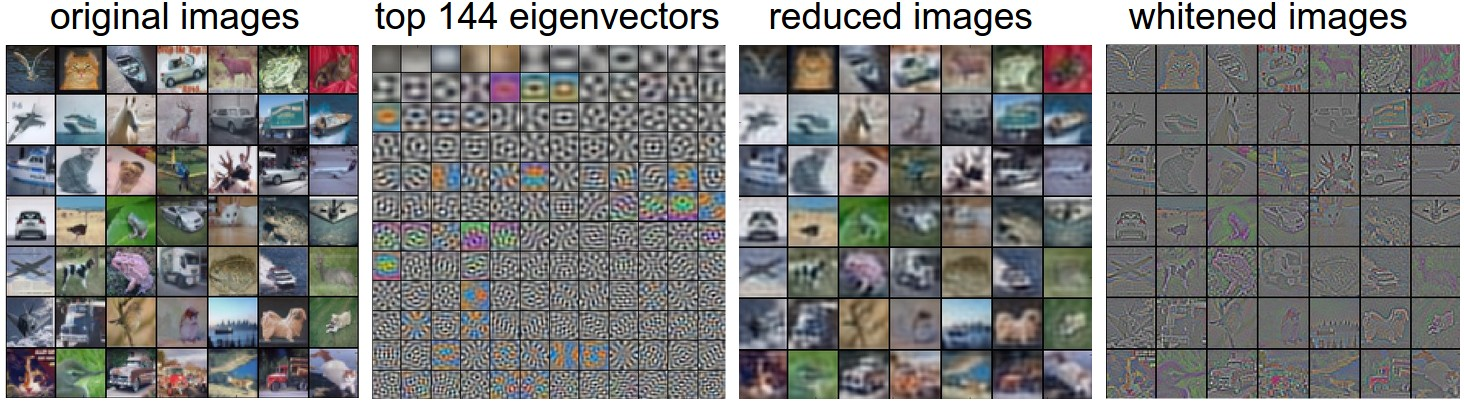我们稍加解释一下，最左边是49张原始图片；左起第2幅图是最3072个特征向量中最top的144个，这144个特征向量包含了绝大多数数据变量信息，而其实它们代表的是图片中低频的信息；左起第3幅图表示PCA降维操作之后的49张图片，使用上面求得的144个特征向量。我们可以观察到图片好像被蒙上了一层东西一样，模糊化了，这也就表明了我们的top144个特征向量捕捉到的都是图像的低频信息，不过我们发现图像的绝大多数信息确实被保留下来了；最右图是whitening的144个数通过乘以U.transpose()[:144,:]还原回图片的样子，有趣的是，我们发现，现在低频信息基本都被滤掉了，剩下一些高频信息被放大呈现。
实际工程中，因为这个部分讲到数据预处理，我们就把基本的几种数据预处理都讲了一遍，但实际卷积神经网中，我们并没有用到去相关和whitening操作。当然，去均值是非常非常重要的，而每个像素维度的归一化也是常用的操作。
特别说明，需要特别说明的一点是，上述的预处理操作，一定都是在训练集上先预算的，然后应用在交叉验证/测试集上的。举个例子，有些同学会先把所有的图片放一起，求均值，然后减掉均值，再把这份数据分作训练集和测试集，这是不对的亲！！！
2.2 权重初始化
我们之前已经看过一个完整的神经网络，是怎么样通过神经元和连接搭建起来的，以及如何对数据做预处理。在训练神经网络之前，我们还有一个任务要做，那就是初始化参数。
错误的想法：全部初始化为0，有些同学说，那既然要训练和收敛嘛，初始值就随便设定，简单一点就全设为0好了。亲，这样是绝对不行的！！！为啥呢？我们在神经网络训练完成之前，是不可能预知神经网络最后的权重具体结果的，但是根据我们归一化后的数据，我们可以假定，大概有半数左右的权重是正数，而另外的半数是负数。但设定全部初始权重都为0的结果是，网络中每个神经元都计算出一样的结果，然后在反向传播中有一样的梯度结果，因此迭代之后的变化情况也都一样，这意味着这个神经网络的权重没有办法差异化，也就没有办法学习到东西。
很小的随机数，其实我们依旧希望初始的权重是较小的数，趋于0，但是就像我们刚刚讨论过的一样，不要真的是0。综合上述想法，在实际场景中，我们通常会把初始权重设定为非常小的数字，然后正负尽量一半一半。这样，初始的时候权重都是不一样的很小随机数，然后迭代过程中不会再出现迭代一致的情况。举个例子，我们可能可以这样初始化一个权重矩阵W=0.0001*np.random.randn(D,H)。这个初始化的过程，使得每个神经元的权重向量初始化为多维高斯中的随机采样向量，所以神经元的初始权重值指向空间中的随机方向。
特别说明：其实不一定更小的初始值会比大值有更好的效果。我们这么想，一个有着非常小的权重的神经网络在后向传播过程中，回传的梯度也是非常小的。这样回传的”信号”流会相对也较弱，对于层数非常多的深度神经网络，这也是一个问题，回传到最前的迭代梯度已经很小了。
方差归一化，上面提到的建议有一个小问题，对于随机初始化的神经元参数下的输出，其分布的方差随着输入的数量，会增长。我们实际上可以通过除以总输入数目的平方根，归一化每个神经元的输出方差到1。也就是说，我们倾向于初始化神经元的权重向量为w = np.random.randn(n) / sqrt(n)，其中n为输入数。
我们从数学的角度，简单解释一下，为什么上述操作可以归一化方差。考虑在激励函数之前的权重w与输入x的内积

s=∑niwixi
$s = \sum_i^n w_i x_i$部分，我们计算一下

s
$s$的方差：

Var(s)=Var(∑inwixi)=∑inVar(wixi)=∑in[E(wi)]2Var(xi)+E[(xi)]2Var(wi)+Var(xi)Var(wi)=∑inVar(xi)Var(wi)=(nVar(w))Var(x)
\begin{align}
\text{Var}(s) &= \text{Var}(\sum_i^n w_ix_i) \\
&= \sum_i^n \text{Var}(w_ix_i) \\
&= \sum_i^n [E(w_i)]^2\text{Var}(x_i) + E[(x_i)]^2\text{Var}(w_i) + \text{Var}(x_i)\text{Var}(w_i) \\
&= \sum_i^n \text{Var}(x_i)\text{Var}(w_i) \\
&= \left( n \text{Var}(w) \right) \text{Var}(x)
\end{align}

注意，这个推导的前2步用到了方差的性质。第3步我们假定输入均值为0，因此

E[xi]=E[wi]=0
$E[x_i] = E[w_i] = 0$。不过这是我们的一个假设，实际情况下并不一定是这样的，比如ReLU单元的均值就是正的。最后一步我们假定

wi,xi
$w_i,x_i$是独立分布。我们想让s的方差和输入x的方差一致，因此我们想让w的方差取值为1/n，又因为我们有公式

Var(aX)=a2Var(X)
$\text{Var}(aX) = a^2\text{Var}(X)$，所以a应该取值为

a=1/n‾‾‾√
$a = \sqrt{1/n}$，numpy里的实现为w = np.random.randn(n) / sqrt(n)。
对于初始化权重还有一些类似的研究和建议，比如说Glorot在论文Understanding the difficulty of training deep feedforward neural networks就推荐使用能满足

Var(w)=2/(nin+nout)
$\text{Var}(w) = 2/(n_{in} + n _{out})$的权重初始化。其中

nin,nout
$n_{in}, n_{out}$是前一层和后一层的神经元个数。而另外一篇比较新的论文Delving Deep into Rectifiers: Surpassing Human-Level Performance on ImageNet Classification，则指出尤其对于ReLU神经元，我们初始化方差应该为2.0/n，也就是w = np.random.randn(n) * sqrt(2.0/n)，目前的神经网络中使用了很多ReLU单元，因此这个设定其实在实际应用中使用最多。
偏移量/bias初始化：相对而言，bias项初始化就简单一些。我们很多时候简单起见，直接就把它们都设为0.在ReLU单元中，有些同学会使用很小的数字(比如0.01)来代替0作为所有bias项的初始值，他们解释说这样也能保证ReLU单元一开始就是被激活的，因此反向传播过程中不会终止掉回传的梯度。不过似乎实际的实验过程中，这个优化并不是每次都能起到作用的，因此很多时候我们还是直接把bias项都初始化为0。
2.3 正则化
在前一节里我们说了我们要通过正则化来控制神经网络，使得它不那么容易过拟合。有几种正则化的类型供选择：
L2正则化，这个我们之前就提到过，非常常见。实现起来也很简单，我们在损失函数里，加入对每个参数的惩罚度。也就是说，对于每个权重

w
$w$，我们在损失函数里加入一项12λw2$\frac{1}{2} \lambda w^2$，其中

λ
$\lambda$是我们可调整的正则化强度。顺便说一句，这里在前面加上1/2的原因是，求导/梯度的时候，刚好变成

λw
$\lambda w$而不是

2λw
$2\lambda w$。L2正则化理解起来也很简单，它对于特别大的权重有很高的惩罚度，以求让权重的分配均匀一些，而不是集中在某一小部分的维度上。我们再想想，加入L2正则化项，其实意味着，在梯度下降参数更新的时候，每个权重以W += -lambda*W的程度被拉向0。L1正则化，这也是一种很常见的正则化形式。在L1正则化中，我们对于每个权重

w
$w$的惩罚项为λ|w|$\lambda |w|$。有时候，你甚至可以看到大神们混着L1和L2正则化用，也就是说加入惩罚项

λ1∣w∣+λ2w2
$\lambda_1 \mid w \mid + \lambda_2 w^2$，L1正则化有其独特的特性，它会让模型训练过程中，权重特征向量逐渐地稀疏化，这意味着到最后，我们只留下了对结果影响最大的一部分权重，而其他不相关的输入(例如『噪声』)因为得不到权重被抑制。所以通常L2正则化后的特征向量是一组很分散的小值，而L1正则化只留下影响较大的权重。在实际应用中，如果你不是特别要求只保留部分特征，那么L2正则化通常能得到比L1正则化更好的效果最大范数约束，另外一种正则化叫做最大范数约束，它直接限制了一个上行的权重边界，然后约束每个神经元上的权重都要满足这个约束。实际应用中是这样实现的，我们不添加任何的惩罚项，就按照正常的损失函数计算，只不过在得到每个神经元的权重向量

w⃗
$\vec{w}$之后约束它满足

∥w⃗ ∥2<c
$\Vert \vec{w} \Vert_2 < c$。有些人提到这种正则化方式帮助他们提高最后的模型效果。另外，这种正则化方式倒是有一点很吸引人：在神经网络训练学习率设定很高的时候，它也能很好地约束住权重更新变化，不至于直接挂掉。Dropout，亲，这个是我们实际神经网络训练中，用的非常多的一种正则化手段，同时也相当有效。Srivastava等人的论文Dropout: A Simple Way to Prevent Neural Networks from Overfitting最早提到用dropout这种方式作为正则化手段。一句话概括它，就是：在训练过程中，我们对每个神经元，都以概率p保持它是激活状态，1-p的概率直接关闭它。
下图是一个3层的神经网络的dropout示意图：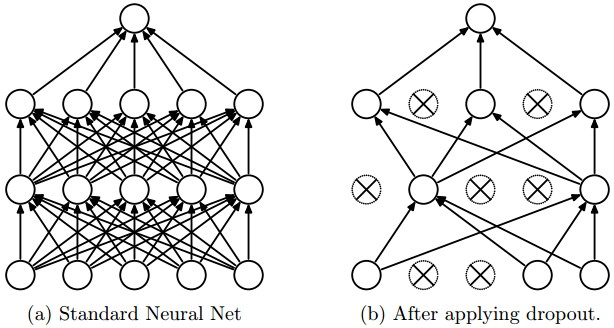可以这么理解，在训练过程中呢，我们对全体神经元，以概率p做了一个采样，只有选出的神经元要进行参数更新。所以最后就从左图的全连接到右图的Dropout过后神经元连接图了。需要多说一句的是，在测试阶段，我们不用dropout，而是直接从概率的角度，对权重配以一个概率值。

简单的Dropout代码如下(这是简易实现版本，但是不建议使用，我们会分析为啥，并在之后给出优化版)：

p = 0.5 # 设定dropout的概率，也就是保持一个神经元激活状态的概率

def train_step(X):
""" X contains the data """

# 3层神经网络前向计算
H1 = np.maximum(0, np.dot(W1, X) + b1)
U1 = np.random.rand(*H1.shape) < p # 第一次Dropout
H1 *= U1 # drop!
H2 = np.maximum(0, np.dot(W2, H1) + b2)
U2 = np.random.rand(*H2.shape) < p # 第二次Dropout
H2 *= U2 # drop!
out = np.dot(W3, H2) + b3

# 反向传播: 计算梯度... (这里省略)
# 参数更新... (这里省略)

def predict(X):
# 加上Dropout之后的前向计算
H1 = np.maximum(0, np.dot(W1, X) + b1) * p
H2 = np.maximum(0, np.dot(W2, H1) + b2) * p
out = np.dot(W3, H2) + b3
上述代码中，在train_step函数中，我们做了2次Dropout。我们甚至可以在输入层做一次dropout。反向传播过程保持不变，除了我们要考虑一下U1,U2
很重要的一点是，大家仔细看predict函数部分，我们不再dropout了，而是对于每个隐层的输出，都用概率p做了一个幅度变换。可以从数学期望的角度去理解这个做法，我们考虑一个神经元的输出为x(没有dropout的情况下)，它的输出的数学期望为

px+(1−p)0
$px + (1-p)0$，那我们在测试阶段，如果直接把每个输出x都做变换

x→px
$x \rightarrow px$，其实是可以保持一样的数学期望的。
上述代码的写法有一些缺陷，我们必须在测试阶段对每个神经的输出都以p的概率输出。考虑到实际应用中，测试阶段对于时间的要求非常高，我们可以考虑反着来，代码实现的时候用inverted dropout，即在训练阶段就做相反的幅度变换/scaling(除以p)，这样在测试阶段，我们可以直接把权重拿来使用，而不用附加很多步用p做scaling的过程。inverted dropout的示例代码如下：
"""
Inverted Dropout的版本，把本该花在测试阶段的时间，转移到训练阶段，从而提高testing部分的速度
"""

p = 0.5 # dropout的概率，也就是保持一个神经元激活状态的概率

def train_step(X):
# f3层神经网络前向计算
H1 = np.maximum(0, np.dot(W1, X) + b1)
U1 = (np.random.rand(*H1.shape) < p) / p # 注意到这个dropout中我们除以p，做了一个inverted dropout
H1 *= U1 # drop!
H2 = np.maximum(0, np.dot(W2, H1) + b2)
U2 = (np.random.rand(*H2.shape) < p) / p # 这个dropout中我们除以p，做了一个inverted dropout
H2 *= U2 # drop!
out = np.dot(W3, H2) + b3

# 反向传播: 计算梯度... (这里省略)
# 参数更新... (这里省略)

def predict(X):
# 直接前向计算，无需再乘以p
H1 = np.maximum(0, np.dot(W1, X) + b1)
H2 = np.maximum(0, np.dot(W2, H1) + b2)
out = np.dot(W3, H2) + b3
对于dropout这个部分如果你有更深的兴趣，欢迎阅读以下文献：  1) 2014 Srivastava 的论文Dropout paper  2) Dropout Training as Adaptive Regularization
bias项的正则化，其实我们在之前的博客中提到过，我们大部分时候并不对偏移量项做正则化，因为它们也没有和数据直接有乘法等交互，也就自然不会影响到最后结果中某个数据维度的作用。不过如果你愿意对它做正则化，倒也不会影响最后结果，毕竟总共有那么多权重项，才那么些bias项，所以一般也不会影响结果。
实际应用中：我们最常见到的是，在全部的交叉验证集上使用L2正则化，同时我们在每一层之后用dropout，很常见的dropout概率为p=0.5，你也可以通过交叉验证去调整这个值。
2.4 损失函数
刚才讨论了数据预处理、权重初始化与正则化相关的问题。现在我们回到训练需要的关键之一：损失函数。对于这么复杂的神经网络，我们也得有一个评估准则去评估预测值和真实结果之间的吻合度，也就是损失函数。神经网络里的损失函数，实际上是计算出了每个样本上的loss，再求平均之后的一个形式，即

L=1N∑iLi
$L = \frac{1}{N} \sum_i L_i$，其中N是训练样本数。
2.4.1 分类问题
分类问题是到目前为止我们一直在讨论的。我们假定一个数据集中每个样本都有唯一一个正确的标签/类别。我们之前提到过有两种损失函数可以使用，其一是SVM的hinge loss:

Li=∑j≠yimax(0,fj−fyi+1)

L_i = \sum_{j\neq y_i} \max(0, f_j - f_{y_i} + 1)

另外一个是Softmax分类器中用到的互熵损失:

Li=−log(efyi∑jefj)

L_i = -\log\left(\frac{e^{f_{y_i}}}{ \sum_j e^{f_j} }\right)

问题：特别多的类别数。当类别标签特别特别多的时候(比如ImageNet包含22000个类别)，层次化的Softmax，它将类别标签建成了一棵树，这样任何一个类别，其实就对应tree的一条路径，然后我们在每个树的结点上都训练一个Softmax以区分是左分支还是右分支。属性分类，上述的两种损失函数都假定，对于每个样本，我们只有一个正确的答案

yi
$y_i$。但是在有些场景下，

yi
$y_i$是一个二值的向量，每个元素都代表有没有某个属性，这时候我们怎么办呢？举个例子说，Instagram上的图片可以看作一大堆hashtag里的一个tag子集，所有一张图片可以有多个tag。对于这种情况，大家可能会想到一个最简单的处理方法，就是对每个属性值都建一个二分类的分类器。比如，对应某个类别的二分类器可能有如下形式的损失函数：

Li=∑jmax(0,1−yijfj)

L_i = \sum_j \max(0, 1 - y_{ij} f_j)

其中的求和是针对有所的类别j，而

yij
$y_{ij}$是1或者-1(取决于第i个样本是否有第j个属性的标签)，打分向量

fj
$f_j$在类别/标签被预测到的情况下为正，其他情况为负。注意到如果正样本有比+1小的得分，或者负样本有比-1大的得分，那么损失/loss就一直在累积。
另外一个也许有效的解决办法是，我们可以对每个属性，都单独训练一个逻辑回归分类器，一个二分类的逻辑回归分类器只有0，1两个类别，属于1的概率为：

P(y=1∣x;w,b)=11+e−(wTx+b)=σ(wTx+b)

P(y = 1 \mid x; w, b) = \frac{1}{1 + e^{-(w^Tx +b)}} = \sigma (w^Tx + b)

又因为0，1两类的概率和为1，所以归属于类别0的概率为

P(y=0∣x;w,b)=1−P(y=1∣x;w,b)
$P(y = 0 \mid x; w, b) = 1 - P(y = 1 \mid x; w,b)$。一个样本在

σ(wTx+b)>0.5
$\sigma (w^Tx + b) > 0.5$的情况下被判定为1，对应sigmoid函数化简一下，对应的是得分

wTx+b>0
$w^Tx +b > 0$。这时候的损失函数可以定义为最大化似然概率的形式，也就是：

Li=∑jyijlog(σ(fj))+(1−yij)log(1−σ(fj))

L_i = \sum_j y_{ij} \log(\sigma(f_j)) + (1 - y_{ij}) \log(1 - \sigma(f_j))

其中标签

yij
$y_{ij}$为1(正样本)或者0(负样本)，而

δ
$\delta$是sigmoid函数。
2.4.2 回归问题
回归是另外一类机器学习问题，主要用于预测连续值属性，比如房子的价格或者图像中某些东西的长度等。对于回归问题，我们一般计算预测值和实际值之间的差值，然后再求L2范数或者L1范数用于衡量。其中对一个样本(一张图片)计算的L2范数损失为：

Li=∥f−yi∥22

L_i = \Vert f - y_i \Vert_2^2

而L1范数损失函数是如下的形式：

Li=∥f−yi∥1=∑j∣fj−(yi)j∣

L_i = \Vert f - y_i \Vert_1 = \sum_j \mid f_j - (y_i)_j \mid

注意：
回归问题中用到的L2范数损失，比分类问题中的Softmax分类器用到的损失函数，更难优化。直观想一想这个问题，一个神经网络最后输出离散的判定类别，比训练它去输出一个个和样本结果对应的连续值，要简单多了。我们前面的博文中提到过，其实Softmax这种分类器，对于输出的打分结果具体值是不怎么在乎的，它只在乎各个类别之间的打分幅度有没有差很多(比如二分类两个类别的得分是1和9，与0.1和0.9)。再一个，L2范数损失健壮性更差一些，异常点和噪声都可能改变损失函数的幅度，而带来大的梯度偏差。一般情况下，对于回归问题，我们都会首先考虑，这个问题能否转化成对应的分类问题，比如说我们把输出值划分成不同的区域(切成一些桶)。举个例子，如果我们要预测一部电影的豆瓣打分，我们可以考虑把得分结果分成1-5颗星，而转化成一个分类问题。如果你觉得问题确实没办法转化成分类问题，那要小心使用L2范数损失：举个例子，在神经网络中，在L2损失函数之前使用dropout是不合适的。

如果我们遇到回归问题，首先要想想，是否完全没有可能把结果离散化之后，把这个问题转化成一个分类问题。

3. 总结
总结一下：
在很多神经网络的问题中，我们都建议对数据特征做预处理，去均值，然后归一化到[-1,1]之间。从一个标准差为

2/n‾‾‾√
$\sqrt{2/n}$的高斯分布中初始化权重，其中n为输入的个数。使用L2正则化(或者最大范数约束)和dropout来减少神经网络的过拟合。对于分类问题，我们最常见的损失函数依旧是SVM hinge loss和Softmax互熵损失。
参考资料与原文
cs231n 神经网络数据预处理正则化与损失函数
展开全文计算机视觉 神经网络 损失函数
• 1 数据预处理 关于数据预处理我们有3个常用的符号，数据矩阵$$X$$，假设其尺寸是$$[N \times D]$$（$$N$$是数据样本的数量，$$D$$是数据的维度）。 1.1 均值减去 均值减法（Mean subtraction）是预处理最常用的形式...

1 数据预处理
关于数据预处理我们有3个常用的符号，数据矩阵$$X$$，假设其尺寸是$$[N \times D]$$（$$N$$是数据样本的数量，$$D$$是数据的维度）。
1.1 均值减去
均值减法（Mean subtraction）是预处理最常用的形式。它对数据中每个独立特征减去平均值，从几何上可以理解为在每个维度上都将数据云的中心都迁移到原点。
在numpy中，该操作可以通过代码$$X-=n p \cdot \operatorname{mean}(X, \text { axis }=0)$$实现。
而对于图像，更常用的是对所有像素都减去一个值，可以用$$\mathrm{X}-=\mathrm{np} \cdot \operatorname{mean}(\mathrm{X})$$实现，也可以在3个颜色通道上分别操作。
1.2 归一化
归一化（Normalization）是指将数据的所有维度都归一化，使其数值范围都近似相等。
有两种常用方法可以实现归一化。
第一种是先对数据做零中心化（zero-centered）处理，然后每个维度都除以其标准差，实现代码为$$\mathrm{X} /=\mathrm{np} . \mathrm{std}(\mathrm{X}, \mathrm{axis}=0)$$。
第二种是对每个维度都做归一化，使得每个维度的最大和最小值是1和-1。这个预处理操作只有在确信不同的输入特征有不同的数值范围（或计量单位）时才有意义，但要注意预处理操作的重要性几乎等同于学习算法本身。在图像处理中，由于像素的数值范围几乎是一致的（都在0-255之间），所以进行这个额外的预处理步骤并不是很必要。
下面直观的看一下均值减法与归一化的效果：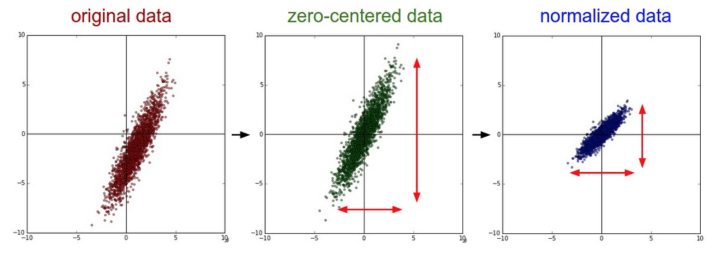上图也是一般数据预处理流程：
左边：原始的2维输入数据。
中间：在每个维度上都减去平均值后得到零中心化数据，现在数据云是以原点为中心的。
右边：每个维度都除以其标准差来调整其数值范围。
红色的线指出了数据各维度的数值范围，在中间的零中心化数据的数值范围不同，但在右边归一化数据中数值范围相同。
1.3 PCA和白化（Whitening）
PCA和白化（Whitening）是另一种预处理形式。
1.3.1 PCA
在这种处理中，先对数据进行零中心化处理，然后计算协方差矩阵，它展示了数据中的相关性结构。
# 假设输入数据矩阵X的尺寸为[N x D]
X -= np.mean(X, axis = 0) # 对数据进行零中心化(重要)
cov = np.dot(X.T, X) / X.shape # 得到数据的协方差矩阵
数据协方差矩阵的第(i, j)个元素是数据第i个和第j个维度的协方差。具体来说，该矩阵的对角线上的元素是方差。
还有，协方差矩阵是对称和半正定的。我们可以对数据协方差矩阵进行SVD（奇异值分解）运算。
U,S,V = np.linalg.svd(cov)
U的列是特征向量，S是装有奇异值的1维数组（因为cov是对称且半正定的，所以S中元素是特征值的平方）。
为了去除数据相关性，将已经零中心化处理过的原始数据投影到特征基准上：
Xrot = np.dot(X,U) # 对数据去相关性
注意U的列是标准正交向量的集合（范式为1，列之间标准正交），所以可以把它们看做标准正交基向量。
因此，投影对应x中的数据的一个旋转，旋转产生的结果就是新的特征向量。如果计算Xrot的协方差矩阵，将会看到它是对角对称的。
np.linalg.svd的一个良好性质是在它的返回值U中，特征向量是按照特征值的大小排列的。
我们可以利用这个性质来对数据降维，只要使用前面的小部分特征向量，丢弃掉那些包含的数据没有方差的维度。 这个操作也被称为主成分分析（ Principal Component Analysis 简称PCA）降维：
Xrot_reduced = np.dot(X, U[:,:100]) # Xrot_reduced 变成 [N x 100]
经过上面的操作，将原始的数据集的大小由[N x D]降到了[N x 100]，留下了数据中包含最大方差的100个维度。
通常使用PCA降维过的数据训练线性分类器和神经网络会达到非常好的性能效果，同时还能节省时间和存储器空间。
1.3.2 白化（Whitening）
白化操作的输入是特征基准上的数据，然后对每个维度除以其特征值来对数值范围进行归一化。
该变换的几何解释是：如果数据服从多变量的高斯分布，那么经过白化后，数据的分布将会是一个均值为零，且协方差相等的矩阵。
该操作的代码如下：
# 对数据进行白化操作:
# 除以特征值
Xwhite = Xrot / np.sqrt(S + 1e-5)
注意分母中添加了1e-5（或一个更小的常量）来防止分母为0。
该变换的一个缺陷是在变换的过程中可能会夸大数据中的噪声，这是因为它将所有维度都拉伸到相同的数值范围，这些维度中也包含了那些只有极少差异性(方差小)而大多是噪声的维度。
在实际操作中，这个问题可以用更强的平滑来解决（例如：采用比1e-5更大的值）。
1.3.3 PCA与白化的差异性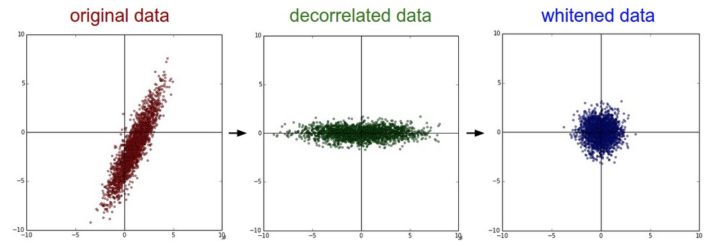左边是二维的原始数据。
中间：经过PCA操作的数据。可以看出数据首先是零中心的，然后变换到了数据协方差矩阵的基准轴上。这样就对数据进行了解相关（协方差矩阵变成对角阵）。
右边：每个维度都被特征值调整数值范围，将数据协方差矩阵变为单位矩阵。从几何上看，就是对数据在各个方向上拉伸压缩，使之变成服从高斯分布的一个数据点分布。
我们可以使用CIFAR-10数据将这些变化可视化出来。
CIFAR-10训练集的大小是50000x3072，其中每张图片都可以拉伸为3072维的行向量。
我们可以计算[3072 x 3072]的协方差矩阵然后进行奇异值分解（比较耗费计算性能），那么经过计算的特征向量看起来是什么样子呢？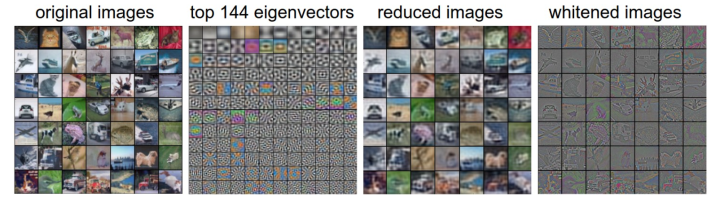最左：一个用于演示的集合，含49张图片。
左二：3072个特征值向量中的前144个。靠前面的特征向量解释了数据中大部分的方差，可以看见它们与图像中较低的频率相关。
第三张是49张经过了PCA降维处理的图片，展示了144个特征向量。
这就是说，展示原始图像是每个图像用3072维的向量，向量中的元素是图片上某个位置的像素在某个颜色通道中的亮度值。而现在每张图片只使用了一个144维的向量，其中每个元素表示了特征向量对于组成这张图片的贡献度。
为了让图片能够正常显示，需要将144维度重新变成基于像素基准的3072个数值。因为U是一个旋转，可以通过乘以U.transpose()[:144,:]来实现，然后将得到的3072个数值可视化。
可以看见图像变得有点模糊了，这正好说明前面的特征向量获取了较低的频率。然而，大多数信息还是保留了下来。
最右：将“白化”后的数据进行显示。
其中144个维度中的方差都被压缩到了相同的数值范围。然后144个白化后的数值通过乘以U.transpose()[:144,:]转换到图像像素基准上。
现在较低的频率（代表了大多数方差）可以忽略不计了，较高的频率（代表相对少的方差）就被夸大了。
1.4 补充
在这里提到PCA和白化主要是为了介绍的完整性，实际上在卷积神经网络中并不会采用这些变换。然而对数据进行零中心化操作还是非常重要的，对每个像素进行归一化也很常见。
进行预处理很重要的一点是：任何预处理策略（比如数据均值）都只能在训练集数据上进行计算，算法训练完毕后再应用到验证集或者测试集上。
例如，如果先计算整个数据集图像的平均值然后每张图片都减去平均值，最后将整个数据集分成训练/验证/测试集，那么这个做法是错误的。
正确的做法是应该先分成训练/验证/测试集，只是从训练集中求图片平均值，然后各个集（训练/验证/测试集）中的图像再减去这个平均值。
2 权重初始化
我们已经看到如何构建一个神经网络的结构并对数据进行预处理，但是在开始训练网络之前，还需要初始化网络的参数。
让我们从应该避免的错误开始。
在训练完毕后，虽然不知道网络中每个权重的最终值应该是多少，但如果数据经过了恰当的归一化的话，就可以假设所有权重数值中大约一半为正数，一半为负数。
这样，一个听起来蛮合理的想法就是把这些权重的初始值都设为0吧，因为在期望上来说0是最合理的猜测。
这个做法错误的！因为如果网络中的每个神经元都计算出同样的输出，然后它们就会在反向传播中计算出同样的梯度，从而进行同样的参数更新。
换句话说，如果权重被初始化为同样的值，神经元之间就失去了不对称性的源头。
2.1 小随机数初始化
因此，权重初始值要非常接近0又不能等于0。解决方法就是将权重初始化为很小的数值，以此来打破对称性。
其思路是：如果神经元刚开始的时候是随机且不相等的，那么它们将计算出不同的更新，并将自身变成整个网络的不同部分。
小随机数权重初始化的实现方法是：W = 0.01 * np.random.randn(D,H)。
其中randn函数是基于零均值和标准差的一个高斯分布来生成随机数的。
根据这个式子，每个神经元的权重向量都被初始化为一个随机向量，而这些随机向量又服从一个多变量高斯分布，这样在输入空间中，所有的神经元的指向是随机的。
也可以使用均匀分布生成的随机数，但是从实践结果来看，对于算法的结果影响极小。
注意：并不是小数值一定会得到好的结果。例如，一个神经网络的层中的权重值很小，那么在反向传播的时候就会计算出非常小的梯度（因为梯度与权重值是成比例的）。这就会很大程度上减小反向传播中的“梯度信号”，在深度网络中，就会出现问题。
除了上述所提到的，上面做法还存在一个问题，即：随着输入数据量的增长，随机初始化的神经元的输出数据的分布中的方差也在增大。
我们可以除以输入数据量的平方根来调整其数值范围，这样神经元输出的方差就归一化到1了。
也就是说，建议将神经元的权重向量初始化为：w = np.random.randn(n) / sqrt(n)。其中n是输入数据的数量。
这样就保证了网络中所有神经元起始时有近似同样的输出分布。实践经验证明，这样做可以提高收敛的速度。
Glorot等在论文"Understanding the difficulty of training deep feedforward neural networks"中作出了类似的分析。
在论文中，作者推荐初始化公式为$$\operatorname{Var}(w)=2 /\left(n_{i n}+n_{o u t}\right)$$，其中$$n_{i n}, n_{o u t}$$是在前一层和后一层中单元的个数。
这是基于妥协和对反向传播中梯度的分析得出的结论。
该主题下最新的一篇论文是："Delving Deep into Rectifiers: Surpassing Human-Level Performance on ImageNet Classification"，作者是He等人。
文中给出了一种针对ReLU神经元的特殊初始化，并给出结论：网络中神经元的方差应该是2.0$$/ n$$。代码为w = np.random.randn(n) * sqrt(2.0/n)。这个形式是神经网络算法使用ReLU神经元时的当前最佳推荐。
2.2 稀疏初始化
另一个处理非标定方差的方法是将所有权重矩阵设为0，但是为了打破对称性，每个神经元都同下一层固定数目的神经元随机连接（其权重数值由一个小的高斯分布生成）。
一个比较典型的连接数目是10个。
2.3 偏置初始化
通常将偏置初始化为0，这是因为随机小数值权重矩阵已经打破了对称性。
对于ReLU非线性激活函数，有研究人员喜欢使用如0.01这样的小数值常量作为所有偏置的初始值，这是因为他们认为这样做能让所有的ReLU单元一开始就激活，这样就能保存并传播一些梯度。
然而，这样做是不是总是能提高算法性能并不清楚（有时候实验结果反而显示性能更差），所以通常还是使用0来初始化偏置参数。
2.4 批量归一化
批量归一化是loffe和Szegedy最近才提出的方法，该方法减轻了如何合理初始化神经网络这个棘手问题带来的头痛。
其做法是让激活数据在训练开始前通过一个网络，网络处理数据使其服从标准高斯分布。
因为归一化是一个简单可求导的操作，所以上述思路是可行的。
在实现层面，应用这个技巧通常意味着全连接层（或者是卷积层，后续会讲）与激活函数之间添加一个BatchNorm层。
在实践中，使用了批量归一化的网络对于不好的初始值有更强的鲁棒性。
最后一句话总结：批量归一化可以理解为在网络的每一层之前都做预处理，只是这种操作以另一种方式与网络集成在了一起。搞定！
3 正则化
3.1 L2正则化
L2正则化可能是最常用的正则化方法了。可以通过惩罚目标函数中所有参数的平方将其实现。
即对于网络中的每个权重$$w$$，向目标函数中增加一个$$\frac{1}{2} \lambda w^{2}$$，其中$$\lambda$$是正则化强度。
前面这个$$\frac{1}{2}$$很常见，是因为加上$$\frac{1}{2}$$后，该式子关于$$w$$梯度就是$$\lambda w$$而不是2$$\lambda w$$了。
L2正则化可以直观理解为它对于大数值的权重向量进行严厉惩罚，倾向于更加分散的权重向量。
在线性分类章节中讨论过，由于输入和权重之间的乘法操作，这样就有了一个优良的特性：使网络更倾向于使用所有输入特征，而不是严重依赖输入特征中某些小部分特征。
最后需要注意在梯度下降和参数更新的时候，使用L2正则化意味着所有的权重都以w += -lambda * W向着0线性下降。
3.2 L1正则化
L1正则化是另一个相对常用的正则化方法。
对于每个w我们都向目标函数增加一个$$\lambda|w|$$。
L1和L2正则化也可以进行组合：$$\lambda_{1}|w|+\lambda_{2} w^{2}$$，这也被称作Elastic net regularizaton。
L1正则化有一个有趣的性质，它会让权重向量在最优化的过程中变得稀疏（即非常接近0）。
也就是说，使用L1正则化的神经元最后使用的是它们最重要的输入数据的稀疏子集，同时对于噪音输入则几乎是不变的了。
相较L1正则化，L2正则化中的权重向量大多是分散的小数字。
在实践中，如果不是特别关注某些明确的特征选择，一般说来L2正则化都会比L1正则化效果好。
3.3 最大范式约束
最大范式约束（Max norm constraints）是给每个神经元中权重向量的量级设定上限，并使用投影梯度下降来确保这一约束。
在实践中，与之对应的是参数更新方式不变，然后要求神经元中的权重向量$$\vec{w}$$必须满足$$\|\vec{w}\|_{2}<c$$这一条件，一般$$c$$值为3或者4。
有研究者发文称在使用这种正则化方法时效果更好。这种正则化还有一个良好的性质，即使在学习率设置过高的时候，网络中也不会出现数值“爆炸”，这是因为它的参数更新始终是被限制着的。
3.4 随机失活
随机失活（Dropout）是一个简单又极其有效的正则化方法。
该方法由Srivastava在论文"Dropout: A Simple Way to Prevent Neural Networks from Overfitting"中提出的，与L1正则化，L2正则化和最大范式约束等方法互为补充。
在训练的时候，随机失活的实现方法是让神经元以超参数$$\mathcal{P}$$的概率被激活或者被设置为0。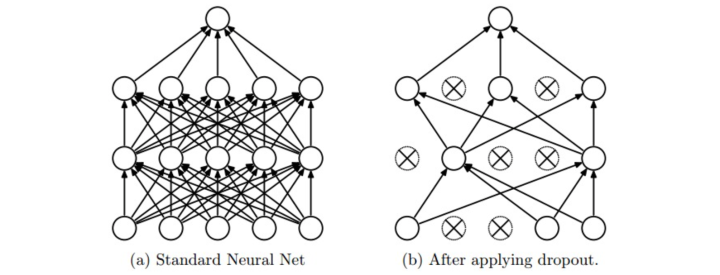上图来源自论文，展示其核心思路。
在训练过程中，随机失活可以被认为是对完整的神经网络抽样出一些子集，每次基于输入数据只更新子网络的参数（然而，数量巨大的子网络们并不是相互独立的，因为它们都共享参数）。
在测试过程中不使用随机失活，可以理解为是对数量巨大的子网络们做了模型集成（model ensemble），以此来计算出一个平均的预测。
一个3层神经网络的普通版随机失活可以用下面代码实现：
""" 普通版随机失活: 不推荐实现 (看下面笔记) """

p = 0.5 # 激活神经元的概率. p值更高 = 随机失活更弱

def train_step(X):
""" X中是输入数据 """

# 3层neural network的前向传播
H1 = np.maximum(0, np.dot(W1, X) + b1)

#np.random.rand()括号里加的是个int型的数，
#而a.shape结果并不是一个int型的数，
#这时候就要在a.shape前面加个*号了。
U1 = np.random.rand(*H1.shape) < p # 第一个随机失活遮罩
H1 *= U1 # drop!
H2 = np.maximum(0, np.dot(W2, H1) + b2)
U2 = np.random.rand(*H2.shape) < p # 第二个随机失活遮罩
H2 *= U2 # drop!
out = np.dot(W3, H2) + b3

# 反向传播:计算梯度... (略)
# 进行参数更新... (略)

def predict(X):
# 前向传播时模型集成
H1 = np.maximum(0, np.dot(W1, X) + b1) * p # 注意：激活数据要乘以p
H2 = np.maximum(0, np.dot(W2, H1) + b2) * p # 注意：激活数据要乘以p
out = np.dot(W3, H2) + b3
在上面的代码中，train_step函数在第一个隐层和第二个隐层上进行了两次随机失活。
在输入层上面进行随机失活也是可以的，为此需要为输入数据$$X$$创建一个二值的遮罩。
反向传播保持不变，但是肯定需要将遮罩U1和U2加入进去。
注意：在predict函数中不进行随机失活，但是对于两个隐层的输出都要乘以$$p$$，调整其数值范围。
这一点非常重要，因为在测试时所有的神经元都能看见它们的输入，因此我们想要神经元的输出与训练时的预期输出是一致的。
以$$p=0.5$$为例，在测试时神经元必须把它们的输出减半，这是因为在训练的时候它们的输出只有一半。
为了理解这点，先假设有一个神经元$$x$$的输出，那么进行随机失活的时候，该神经元的输出就是$$p x+(1-p) 0$$，这是有$$1-p$$的概率神经元的输出为0。
在测试时神经元总是激活的，就必须调整$$x \rightarrow p x$$来保持同样的预期输出。
在测试时会在所有可能的二值遮罩（也就是数量庞大的所有子网络）中迭代并计算它们的协作预测，进行这种减弱的操作也可以认为是与之相关的。
上述操作不好的性质是必须在测试时对激活数据要按照$$p$$进行数值范围调整。
既然测试性能如此关键，实际更倾向使用反向随机失活（inverted dropout），它是在训练时就进行数值范围调整，从而让前向传播在测试时保持不变。
这样做还有一个好处，无论你决定是否使用随机失活，预测方法的代码可以保持不变。反向随机失活的代码如下：
"""
反向随机失活: 推荐实现方式.
在训练的时候drop和调整数值范围，测试时不做任何事.
"""

p = 0.5 # 激活神经元的概率. p值更高 = 随机失活更弱

def train_step(X):
# 3层neural network的前向传播
H1 = np.maximum(0, np.dot(W1, X) + b1)
U1 = (np.random.rand(*H1.shape) < p) / p # 第一个随机失活遮罩. 注意/p!
H1 *= U1 # drop!
H2 = np.maximum(0, np.dot(W2, H1) + b2)
U2 = (np.random.rand(*H2.shape) < p) / p # 第二个随机失活遮罩. 注意/p!
H2 *= U2 # drop!
out = np.dot(W3, H2) + b3

# 反向传播:计算梯度... (略)
# 进行参数更新... (略)

def predict(X):
# 前向传播时模型集成
H1 = np.maximum(0, np.dot(W1, X) + b1) # 不用数值范围调整了
H2 = np.maximum(0, np.dot(W2, H1) + b2)
out = np.dot(W3, H2) + b3
3.5 偏置正则化
在线性分类器的章节中介绍过，对于偏置参数的正则化并不常见，因为它们在矩阵乘法中和输入数据并不产生互动，所以并不需要控制其在数据维度上的效果。
然而在实际应用中（使用了合理数据预处理的情况下），对偏置进行正则化也很少会导致算法性能变差。
这可能是因为相较于权重参数，偏置参数实在太少，所以分类器需要它们来获得一个很好的数据损失，那么还是能够承受的。
3.6 每层正则化
对于不同的层进行不同强度的正则化很少见（可能除了输出层以外），关于这个思路的相关文献也很少。
在实际中，通过交叉验证获得一个全局使用的L2正则化强度是比较常见的。在使用L2正则化的同时在所有层后面使用随机失活也很常见。
$$p$$值一般默认设为0.5，也可能在验证集上调参。
4 损失函数
我们已经讨论过损失函数的正则化损失部分，它可以看做是对模型复杂程度的某种惩罚。
损失函数的第二个部分是数据损失，它是一个有监督学习问题，用于衡量分类算法的预测结果（即分类评分）和真实标签结果之间的一致性。
数据损失是对所有样本的数据损失求平均。也就是说，$$L=\frac{1}{N} \sum_{i} L_{i}$$中，$$N$$是训练集数据的样本数。
让我们把神经网络中输出层的激活函数简写为$$f=f\left(x_{i} ; W\right)$$。
4.1 分类问题
分类问题是我们一直讨论的。
在该问题中，假设有一个装满样本的数据集，每个样本都有一个唯一的正确标签（是固定分类标签之一）。
在这类问题中，一个最常见的损失函数就是SVM（是Weston Watkins 公式）：

$L_{i}=\sum_{j \neq y_{i}} \max \left(0, f_{j}-f_{y_{i}}+1\right)$

之前简要提起过，有些学者的论文中指出平方折叶损失（即使用$$\max \left(0, f_{j}-f_{y_{i}}+1\right)^{2}$$）算法的结果会更好。
第二个常用的损失函数是Softmax分类器，它使用交叉熵损失：

$L_{i}=-\log \left(\frac{e^{f_{y_{i}}}}{\sum_{j} e^{f_{j}}}\right)$

当标签集非常庞大（例如字典中的所有英语单词，或者ImageNet中的22000种分类），就需要使用分层Softmax（Hierarchical Softmax）了。
分层softmax将标签分解成一个树。每个标签都表示成这个树上的一个路径，这个树的每个节点处都训练一个Softmax分类器来在左和右分枝之间做决策。
树的结构对于算法的最终结果影响很大，而且一般需要具体问题具体分析。
上面两个损失公式的前提，都是假设每个样本只有一个正确的标签$$\mathcal{y}_{i}$$。但是如果$$\mathcal{y}_{i}$$是一个二值向量，每个样本可能有，也可能没有某个属性，而且属性之间并不相互排斥呢？
比如在Instagram上的图片，就可以看成是被一个巨大的标签集合中的某个子集打上标签，一张图片上可能有多个标签。
在这种情况下，一个明智的方法是为每个属性创建一个独立的二分类的分类器。
例如，针对每个分类的二分类器会采用下面的公式：

$L_{i}=\sum_{j} \max \left(0,1-y_{i j} f_{j}\right)$

上式中，求和是对所有分类$$j$$，$$y_{i j}$$的值为1或者-1，具体根据第i个样本是否被第j个属性打标签而定，当该类别被正确预测并展示的时候，分值向量$$f_{j}$$为正，其余情况为负。
可以发现，当一个正样本的得分小于+1，或者一个负样本得分大于-1的时候，算法就会累计损失值。
另一种方法是对每种属性训练一个独立的逻辑回归分类器。
二分类的逻辑回归分类器只有两个分类（0，1），其中对于分类1的概率计算为：

$P(y=1 | x ; w, b)=\frac{1}{1+e^{-\left(w^{T} x+b\right)}}=\sigma\left(w^{T} x+b\right)$

因为类别0和类别1的概率和为1，所以类别0的概率为：

$P(y=0 | x ; w, b)=1-P(y=1 | x ; w, b)$

这样，如果$$\sigma\left(w^{T} x+b\right)>0.5$$或者$$w^{T} x+b>0$$，那么样本就要被分类成为正样本（y=1）。
然后损失函数最大化这个对数似然函数，问题可以简化为：

$L_{i}=\sum_{j} y_{i j} \log \left(\sigma\left(f_{j}\right)\right)+\left(1-y_{i j}\right) \log \left(1-\sigma\left(f_{j}\right)\right)$

上式中，假设标签$$y_{i j}$$非0即1，$$\sigma( .)$$就是sigmoid函数。上面的公式看起来吓人，但是$$f$$的梯度实际上非常简单：$$\frac{\partial L_{i}}{\partial f_{j}}=y_{i j}-\sigma\left(f_{j}\right)$$。
4.2 回归问题
回归问题是预测实数的值的问题，比如预测房价，预测图片中某个东西的长度等。
对于这种问题，通常是计算预测值和真实值之间的损失。然后用L2平方范式或L1范式度量差异。
对于某个样本，L2范式计算如下：

$L_{i}=\left\|f-y_{i}\right\|_{2}^{2}$

之所以在目标函数中要进行平方，是因为梯度算起来更加简单。因为平方是一个单调运算，所以不用改变最优参数。
L1范式则是要将每个维度上的绝对值加起来：

$L_{i}=\left\|f-y_{i}\right\|_{1}=\sum_{j}\left|f_{j}-\left(y_{i}\right)_{j}\right|$

在上式中，如果有多个数量被预测了，就要对预测的所有维度的预测求和，即$$\sum_{j}$$。
观察第i个样本的第j维，用$$\delta_{i j}$$表示预测值与真实值之间的差异。
关于该维度的梯度（也就是$$\partial L_{i} / \partial f_{j}$$）能够轻松地通过被求导为L2范式的$$\delta_{i j}$$或者$$\operatorname{sign}\left(\delta_{i j}\right)$$。
这就是说，评分值的梯度要么与误差中的差值直接成比例，要么是固定的并从差值中继承sign。
注意：L2损失比起较为稳定的Softmax损失来，其最优化过程要困难很多。
直观而言，它需要网络具备一个特别的性质，即对于每个输入（和增量）都要输出一个确切的正确值。
而在Softmax中就不是这样，每个评分的准确值并不是那么重要：只有当它们量级适当的时候，才有意义。
还有，L2损失鲁棒性不好，因为异常值可以导致很大的梯度。所以在面对一个回归问题时，先考虑将输出变成二值化是否真的不够用。
例如，如果对一个产品的星级进行预测，使用5个独立的分类器来对1-5星进行打分的效果一般比使用一个回归损失要好很多。
分类还有一个额外优点，就是能给出关于回归的输出的分布，而不是一个简单的毫无把握的输出值。
如果确信分类不适用，那么使用L2损失吧，但是一定要谨慎：L2非常脆弱，在网络中使用随机失活（尤其是在L2损失层的上一层）不是好主意。
4.3 结构化损失
结构化损失是指标签可以是任意的结构，例如图表、树或者其他复杂物体的情况。
通常这种情况还会假设结构空间非常巨大，不容易进行遍历。
结构化SVM背后的基本思想就是在正确的结构$$\mathcal{y}_{i}$$和得分最高的非正确结构之间画出一个边界。
解决这类问题，并不是像解决一个简单无限制的最优化问题那样使用梯度下降就可以了，而是需要设计一些特殊的解决方案，这样可以有效利用对于结构空间的特殊简化假设。

转载于:https://www.cnblogs.com/Terrypython/p/11013185.html
展开全文• 这一节我们讲一讲输入数据预处理正则化以及损失函数设定的一些事情。 2. 数据与网络的设定 前一节提到前向计算涉及到的组件(主要是神经元)设定。神经网络结构和参数设定完毕之后，我们就得到得分函数/score ...深度学习 计算机视觉 图像分类 损失函数...